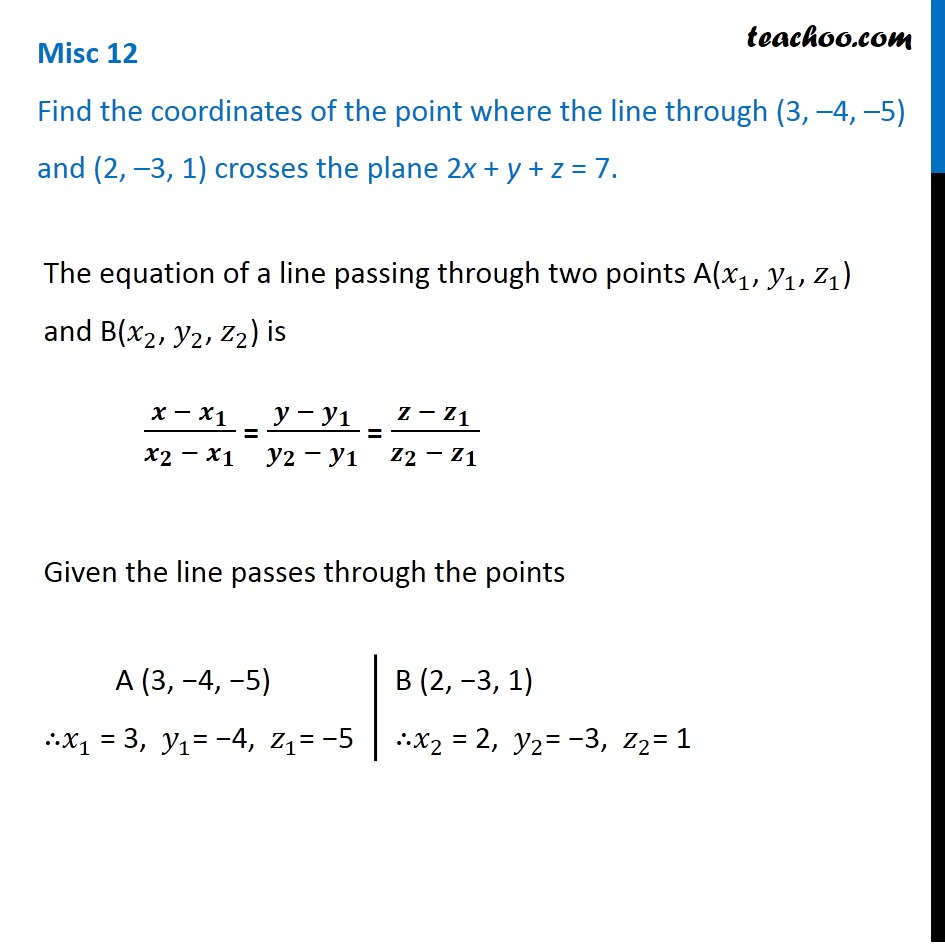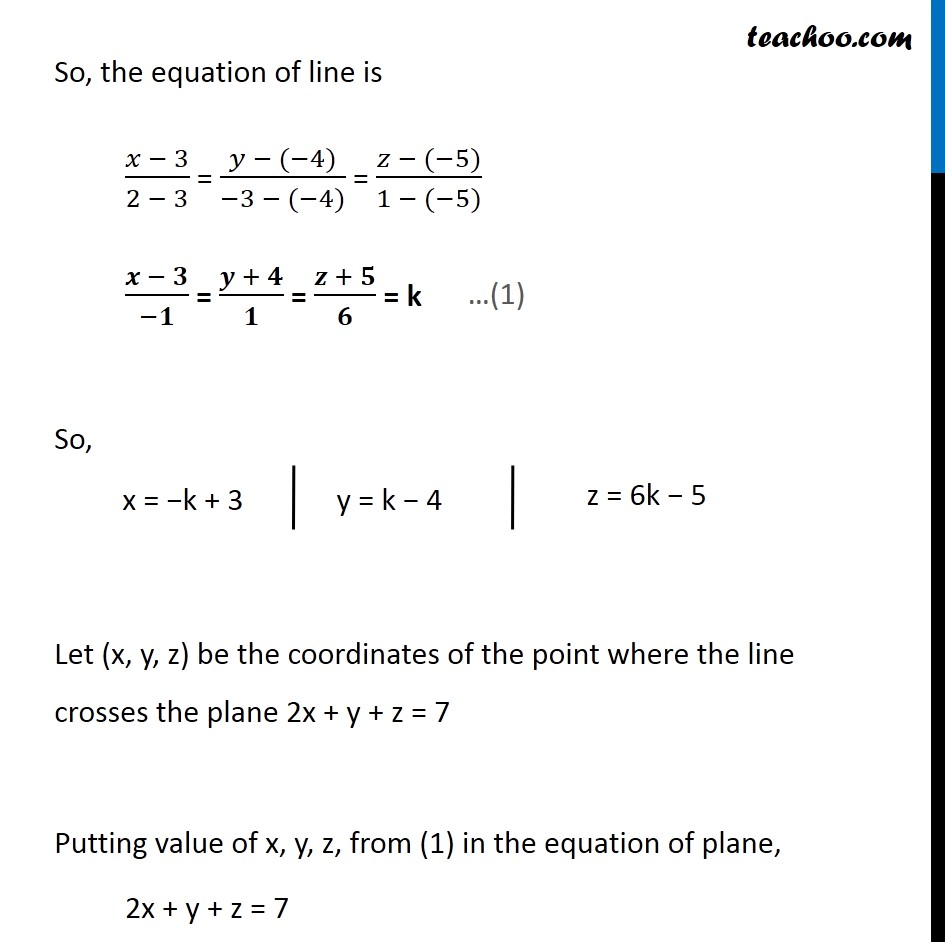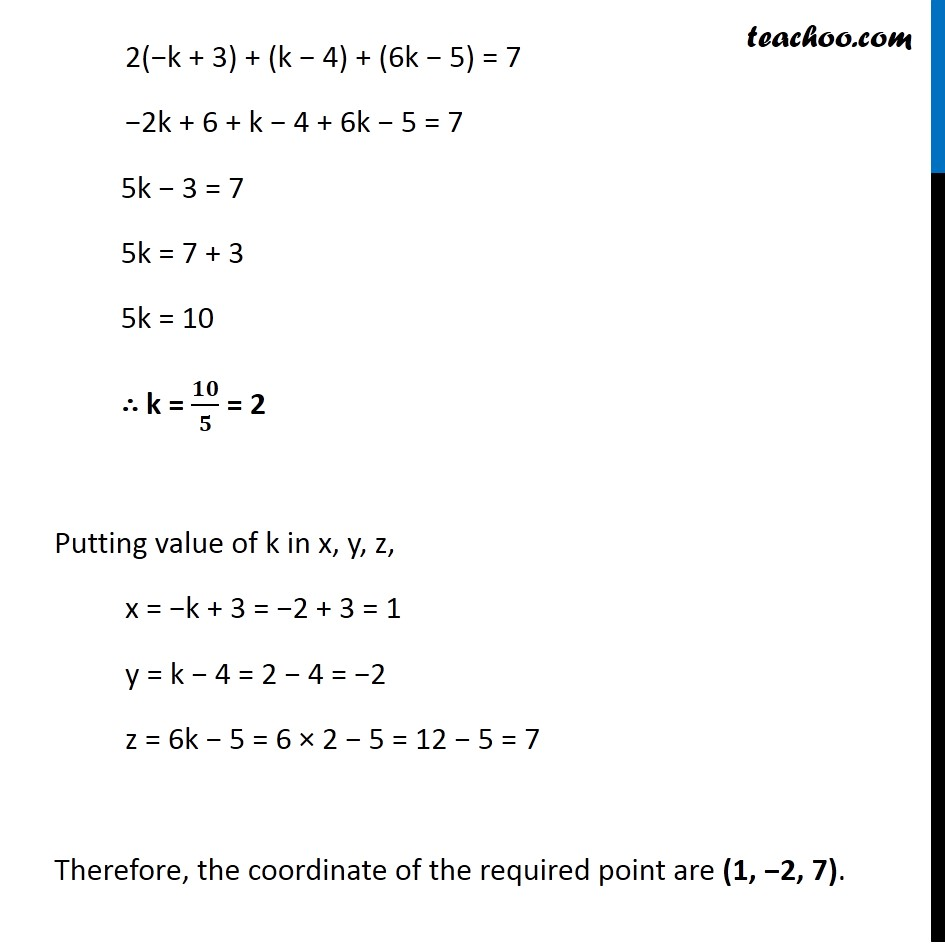Miscellaneous

Chapter 11 Class 12 Three Dimensional Geometry
Serial order wiseLearn in your speed, with individual attention - Teachoo Maths 1-on-1 Class

### Transcript

Question 8 Find the coordinates of the point where the line through (3, –4, –5) and (2, –3, 1) crosses the plane 2x + y + z = 7. The equation of a line passing through two points A(𝑥_1, 𝑦_1, 𝑧_1) and B(𝑥_2, 𝑦_2, 𝑧_2) is (𝒙 − 𝒙_𝟏)/(𝒙_𝟐 − 𝒙_𝟏 ) = (𝒚 − 𝒚_𝟏)/(𝒚_𝟐 − 𝒚_𝟏 ) = (𝒛 − 𝒛_𝟏)/(𝒛_𝟐 − 𝒛_𝟏 ) Given the line passes through the points A (3, −4, −5) ∴𝑥_1 = 3, 𝑦_1= −4, 𝑧_1= −5 B (2, −3, 1) ∴𝑥_2 = 2, 𝑦_2= −3, 𝑧_2= 1 So, the equation of line is (𝑥 − 3)/(2 − 3) = (𝑦 − (−4))/(−3 − (−4)) = (𝑧 − (−5))/(1 − (−5)) (𝒙 − 𝟑)/(−𝟏) = (𝒚 + 𝟒)/𝟏 = (𝒛 + 𝟓)/𝟔 = k So, Let (x, y, z) be the coordinates of the point where the line crosses the plane 2x + y + z = 7 Putting value of x, y, z, from (1) in the equation of plane, 2x + y + z = 7 x = −k + 3 2(−k + 3) + (k − 4) + (6k − 5) = 7 −2k + 6 + k − 4 + 6k − 5 = 7 5k − 3 = 7 5k = 7 + 3 5k = 10 ∴ k = 𝟏𝟎/𝟓 = 2 Putting value of k in x, y, z, x = −k + 3 = −2 + 3 = 1 y = k − 4 = 2 − 4 = −2 z = 6k − 5 = 6 × 2 − 5 = 12 − 5 = 7 Therefore, the coordinate of the required point are (1, −2, 7).